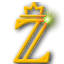TU-Berlin → Inst. f. Mathematik → Algebra und Zahlentheorie → KASH → Documentation → Reference ManualKASH3 Reference Manual
search

Ideals

### Description

In KASH3 an ideal is an object which is, like an algebraic number, defined over a certain order. There are many ways to create an ideal in KASH3. The most basic one is to use the function 'Ideal'. The sum and the difference of two ideals are the smallest ideals which contain both operands. The product of two ideals is the ideal formed by all products of an element of the first ideal with an element of the second one. KASH3 can also handle fractional ideals (a fractional ideal is an integral ideal divided by a certain non--zero integer). This feature allows ideals to be inverted if the underlying order is the maximal one (remember that in a Dedekind ring the fractional ideals form a group under multiplication).

Built: Mon Nov 14 21:12:39 UTC 2005 on mack
The KANT Group